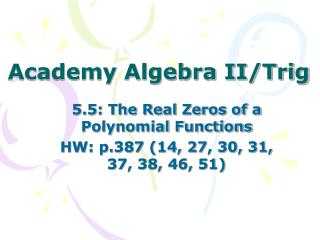DownloadDownload Presentation# Academy Algebra II/Trig

Download Presentation## Academy Algebra II/Trig

- - - - - - - - - - - - - - - - - - - - - - - - - - - E N D - - - - - - - - - - - - - - - - - - - - - - - - - - -
##### Presentation Transcript

1. Academy Algebra II/Trig 5.5: The Real Zeros of a Polynomial Functions HW: p.387 (14, 27, 30, 31, 37, 38, 46, 51)

2. Factor Theorem: If f(c) = 0, then x-c is a factor of f(x).Using the factor theorem, determine whether the function has the factor given. Note: You could also divide to check.

3. List all possible rational zeros using the rational zero theorem. Let f be a polynomial of degree 1 or higher of the form: The possible rational zeros =

4. List all possible rational zeros using the rational zero theorem. Example: List the possible rational zeros for the function: Factors of the constant: Factors of the leading coefficient: Possible rational zeros: Possible rational zeros:

5. Descartes’ Rule of Signs # of positive real zeros: # of sign changes for f(x) or an even integer less than the sign change. # of negative real zeros: # of sign changes for f(-x) or an even integer less than the sign change.

6. Use Descartes’ Rule of Signs to discuss the real zeros of the polynomial.

7. Find the zeros of a polynomial function. List the possible rational zeros of the function. Use Descartes’ Rule of Signs to determine the possible number of positive and negative zeros. Test the zeros using division. (Since the zeros are x-intercepts, when you divide you should end up with a remainder of zero.) Factor if you get a factorable polynomial.

8. Find all real zeros of the function.

9. Find all real zeros of the function.

10. Find all real zeros of the function.

11. Academy Algebra II/Trig 5.5: The Real Zeros of a Polynomial Functions HW: p.387-388 (55-58, 67, 70) Test: 4.3-4.5, 5.1, 5.5, 5.6

12. Find the real solutions of the equation.

13. Find the real solutions of the equation.

14. Academy Algebra II/Trig 5.6: Complex Zeros, Fundamental Theorem of Algebra

15. Find the zeros of the polynomial function. f(x) = 3x4 + 5x3 + 25x2 + 45x – 18

16. Do Now: Solve the equation. x3 + 5x2 + 4x + 20 = 0

17. Academy Algebra II/Trig 5.6: Complex Zeros, Fundamental Theorem of Algebra HW: p.394 (16, 20, 21, 31-33, 40) Test: 4.3-4.5, 5.1, 5.5, 5.6

18. Fundamental Theorem of Algebra If f(x) is a polynomial with degree of n (where n>0), then the equation f(x) = 0 has at least one solution. Corollary: The equation f(x) = 0 has exactly n solutions provided you count multiple solutions.

19. Conjugates Theorem If is an imaginary zero of function f, then is also a zero. If is a zero of function f where is irrational, then is also a zero.

20. Write a polynomial function f of least degree that has rational coefficients, a leading coefficient of 1, & the given zeros. 1.) -1, 2, 4 2.) 4, 2i,

21. Write a polynomial function f with the given degree, rational coefficients, a leading coefficient of 1, & the given zeros. 3.) degree 4; zeros: 3, multiplicity 2; i

22. Find all zeros of the polynomial function. f(x) = x4 - 13x2 + 36